### Home > CALC > Chapter 5 > Lesson 5.5.1 > Problem5-160

5-160.
1. Bob's cat Alice does wind sprints up and down the hallway of his apartment. Suppose that t minutes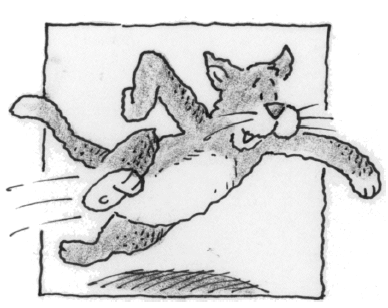into her wind sprints, Alice's velocity (in ft/min) is given by the formula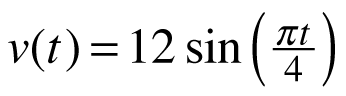. Homework Help ✎

1. What is Alice's acceleration at t = 6 seconds?

2. What is Alice's displacement between t = 4 and t = 24?

3. What is the total distance traveled by Alice between t = 2 and t = 8?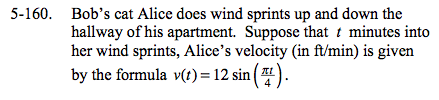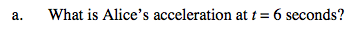Acceleration is the derivative of velocity.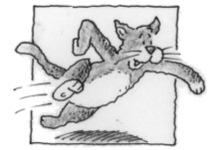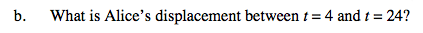Displacement is the area under a velocity graph. Total distance is the area under a speed graph.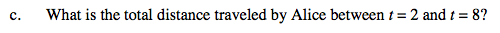Refer to the hint in part (b).